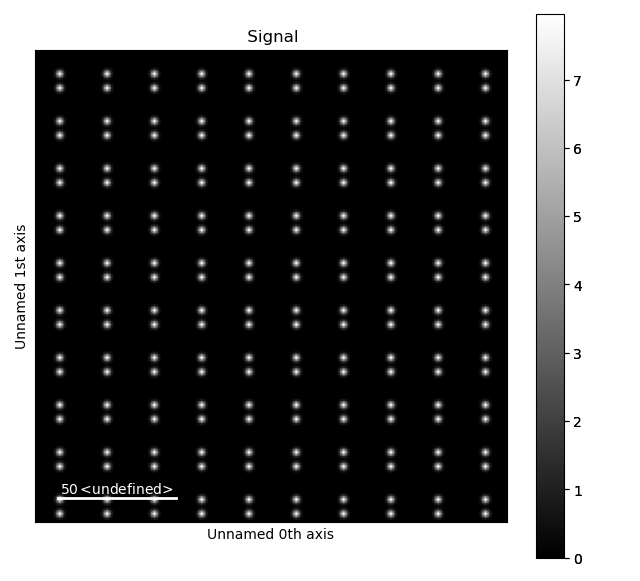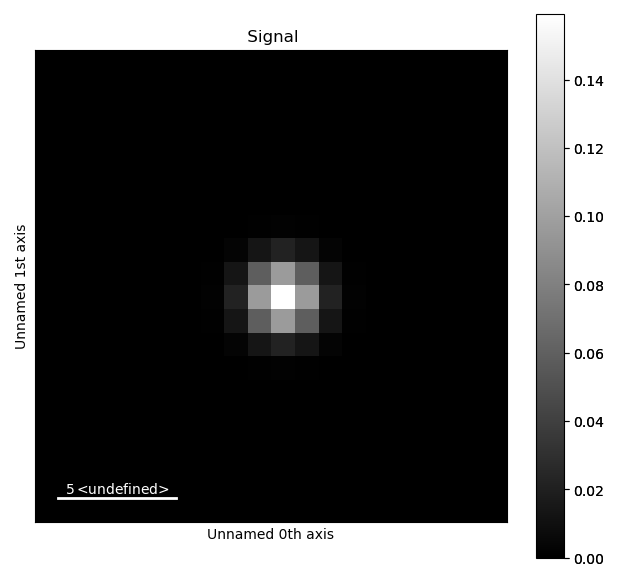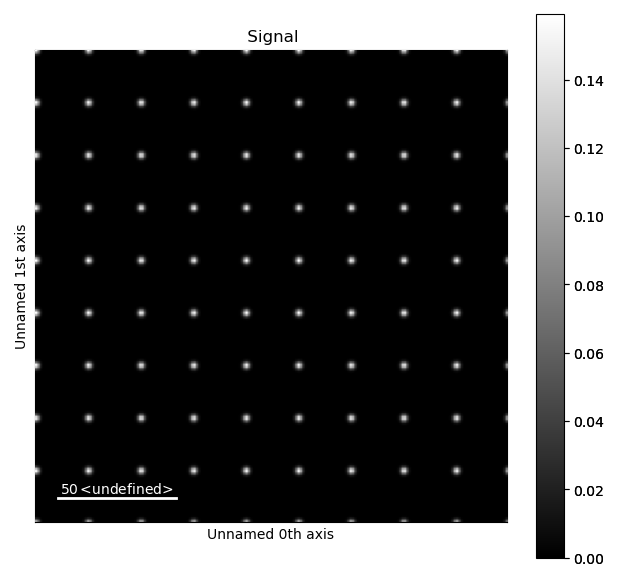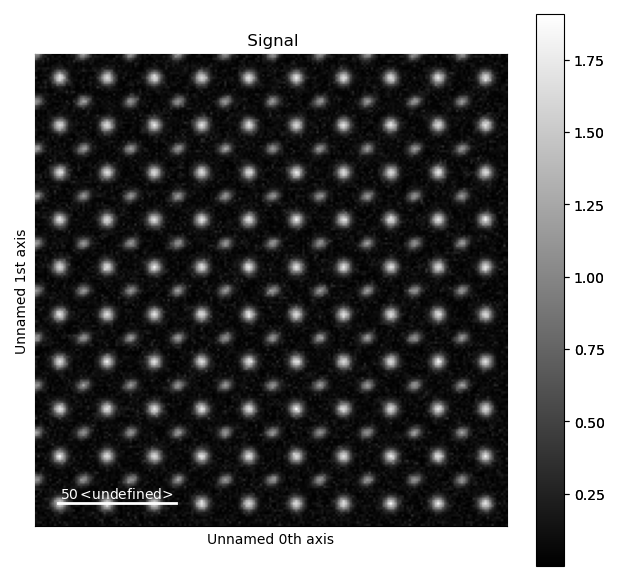# Making datasets for testing¶

The functionality for generating specific test data is useful for

1. Testing processing tools on known standards

2. Finding parameter sensitivity for processing tools

3. Easily generate example datasets

4. Use in unit tests for Atomap

The `atomap.dummy_data` module contains several pre-generated structures:

```>>> import atomap.api as am
>>> s = am.dummy_data.get_dumbbell_signal()
>>> s.plot()
```A full list of these pre-generated structures can be found in the API documentation for the dummy data module: `atomap.dummy_data`

In addition, your own structures can be generated using the `atomap.testing_tools.MakeTestData` class. This tutorial will show how to do this.

The class is initialized by specifying the size of the image, using `image_x` and `image_y`.

```>>> import atomap.testing_tools as tt
>>> t1 =  tt.MakeTestData(image_x=20, image_y=20)
>>> t1.signal.plot()
```To a MakeTestData object you can add single atoms, or lists of atoms. Note that the `x` and `y` arrays needs to be flattened first.

```>>> import numpy as np
>>> t2 = tt.MakeTestData(200,200)
>>> x, y = np.mgrid[0:200:10j, 0:200:10j]
>>> x, y = x.flatten(), y.flatten()
>>> t2.signal.plot()
```The key is to make lists of the x and y positions of the atoms in a sublattice. In the above example, the j means that 10 positions will be distributed between 0 and 200. Below, the exact separation between the positions is set. You can add many sublattices, and for each sublattice you can set the properties of the Gaussian used to model the atoms.

```>>> t3 = tt.MakeTestData(200, 200)
>>> x, y = np.mgrid[0:200:20, 0:200:20]
>>> x, y = x.flatten(), y.flatten()
>>> t3.add_atom_list(x, y,sigma_x=2, sigma_y=1.5, amplitude=20, rotation=0.4)

>>> x, y = np.mgrid[10:200:20, 10:200:20]
>>> x, y = x.flatten(), y.flatten()
>>> t3.signal.plot()
```In the last example, image noise is added. The image noise is currently Gaussian distributed, and both the standard deviation and expectation value of the noise (mu) can be set. By default, mu=0.

It is also possible to make sublattice and atom lattice objects:

```>>> sublattice3 = t3.sublattice
>>> atom_lattice3 = t3.atom_lattice
```

## Large amount of atoms¶

Generating test signals with a large amount of atoms (2000+) can take a very long time. However, sublattice objects can be generated quickly if the image is not needed. This is done by using `sublattice_generate_image=False`:

```>>> testdata = tt.MakeTestData(1000, 1000, sublattice_generate_image=False)
>>> x, y = np.mgrid[0:1000:150j, 0:1000:150j]
>>> x, y = x.flatten(), y.flatten()